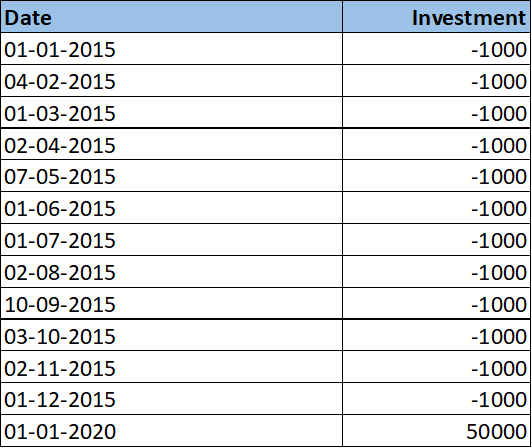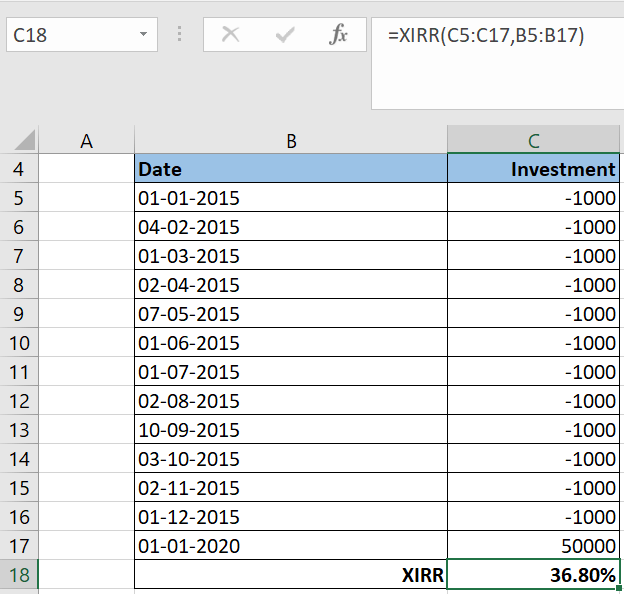### Understanding Extended Internal Rate of Return (XIRR)

XIRR, standing for Extended Internal Rate of Return, is an essential tool to calculate XIRR and evaluate investment performance, akin to CAGR. It excels in assessing returns from investments made at various times throughout a period.

### The Practicality of XIRR in Diverse Investments

Take systematic investment plans (SIP) for instance, where you make multiple investments throughout the year. Some are regular, like monthly contributions, while others are sporadic, like lump-sum bonus investments. XIRR is an ideal tool for measuring returns on such periodic investments.

### XIRR: A Detailed Analysis

In this post, we’ll dive deep into XIRR, compare it with CAGR, and guide you on how to calculate it using an Excel sheet.

Related Read: Calculating CAGR in Excel

### Differentiating XIRR from CAGR

Let’s say you invest 1000 monthly for 12 months, and this investment grows to 50,000 in 5 years. To calculate the CAGR:

CAGR = (50000/12000)1/5 -1 = 33.03%

But here’s a crucial aspect:

CAGR assumes all investments span 60 months (5 years), which isn’t accurate. Your first investment indeed matures in 60 months, but subsequent investments mature in lesser time, reducing month by month.

Why XIRR Makes Sense Here

For such staggered investments, it’s more logical to compute individual CAGRs and amalgamate them into a single rate, known as XIRR. For our example, XIRR calculates to 36.8%.

### Calculating XIRR in Excel

XIRR is straightforward to calculate in Excel, which even has a built-in function for it. This function employs the Newton Raphson method to derive the aggregated CAGR, or XIRR, for each investment.

To compute XIRR, log each investment and its date in Excel. Mark investments as negative and redemptions as positive.

See below:In this example, a monthly investment of 1000 is made throughout 2015, culminating in a 50,000 redemption after 5 years in 2020.

This final figure can also represent the current investment value, not just a redemption amount.

Using the XIRR Formula in Excel

To calculate XIRR, use the following formula:

=XIRR (values, dates, [guess])

Values – Cells containing investment or redemption amounts

Dates – Corresponding dates of investment or redemption

Guess – [optional] An estimated expected IRR. Defaults to 10%.

Using this formula, XIRR is calculated as 36.8%. See below:XIRR Calculation Excel Sheet

This Excel template is invaluable for accurately calculating returns on systematic investments in mutual funds or stocks.### Price Momentum Oscillator (PMO) Amibroker AFL CodedexterSeptember 20, 2023### How to calculate Stop Loss in an Excel Sheet?dexterNovember 25, 2023### Basic Terms to Learn for Aspiring Online TradersdexterSeptember 20, 2023